﻿ 基于距离配对排序的支持向量预选取算法 Pre-Selection of Support Vectors Base on Distance Pairing Sorting

Vol. 09  No. 02 ( 2020 ), Article ID: 34225 , 9 pages
10.12677/AAM.2020.92023

Pre-Selection of Support Vectors Base on Distance Pairing Sorting

Chengzhi Han*, Entao Zheng, Guochun Ma#

College of Science, Hangzhou Normal University, Hangzhou Zhejiang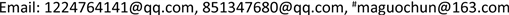Received: Jan. 30th, 2020; accepted: Feb. 12th, 2020; published: Feb. 19th, 2020ABSTRACT

Support Vector Machine is a binary classification method based on statistical learning theory. The Sequential minimal optimization algorithm is an efficient algorithm developed for the dual problem of SVM. In the data training process, support vectors play a decisive role in the determination of separation hyperplane. However, support vectors are only a small part of the original sample set and distributed in the boundary of two types of data. If a boundary vector set containing most support vectors is used to replace the original sample set for training, the training time can be shortened and the classification speed can be improved on the premise of guaranteeing the classification accuracy. Pre-selection of support vector is difficult. In order to solve this problem, this paper proposes a support vector pre-selection algorithm based on distance pairing sort. The experimental results show that the proposed algorithm can effectively pre-select the set of boundary vectors containing support vectors.

Keywords:Support Vector Machine, Support Vector, Pre-Selection, The Boundary Vector Set, Distance Pairing Sorting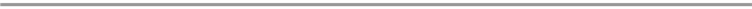1. 引言

2. 支持向量机简介(1)

$f\left(x\right)=sign\left(\underset{i=1}{\overset{N}{\sum }}{y}_{i}{\alpha }_{i}^{\ast }K\left(x,{x}_{i}\right)+{b}^{*}\right).$ (2)

3. 基于距离配对排序支持向量预选取算法

3.1. 样本距离

$d\left({x}_{1},{x}_{2}\right)=\sqrt{K\left({x}_{1},{x}_{1}\right)-2K\left({x}_{1},{x}_{2}\right)+K\left({x}_{2},{x}_{2}\right)},$ (3)

$d\left({x}_{1},{x}_{2}\right)={‖{x}_{1}-{x}_{2}‖}_{2}=\sqrt{\underset{i=1}{\overset{n}{\sum }}{\left({x}_{1}^{i}-{x}_{2}^{i}\right)}^{2}},$ (4)

3.2. 边界向量集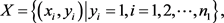(5)

${X}^{\prime }=\left\{\left({{x}^{\prime }}_{j},{y}_{j}\right)|{y}_{j}=-1,j=1,2,\cdots ,{n}_{2}\right\},$ (6)(7)

$l=⌈{n}_{1}a⌉,$ (8)

${D}^{\prime }$ 经抽取之后的集合用 ${D}^{″}$ 来表示，即：

${D}^{″}=\left({d}_{1},{d}_{2},\cdots ,{d}_{l}\right),$ (9)

$S=\left\{\left({x}_{{k}_{1}},{{x}^{\prime }}_{{k}_{1}}\right),\left({x}_{{k}_{2}},{{x}^{\prime }}_{{k}_{2}}\right),\cdots ,\left({x}_{{k}_{l}},{{x}^{\prime }}_{{k}_{l}}\right)\right\}.$ (10)

$A=\left\{{x}_{{k}_{1}},{x}_{{k}_{2}},\cdots ,{x}_{{k}_{l}}\right\}\cup \left\{{{x}^{\prime }}_{{k}_{1}},{{x}^{\prime }}_{{k}_{2}},\cdots ,{{x}^{\prime }}_{{k}_{l}}\right\}.$ (11)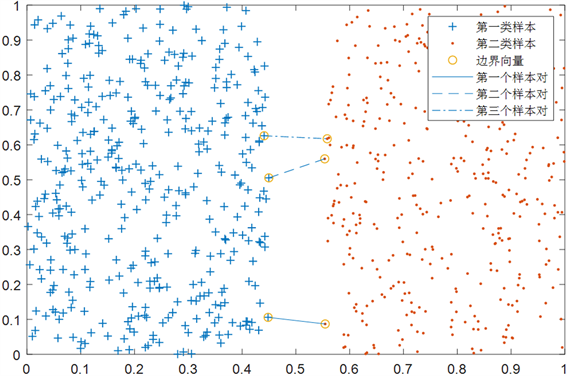Figure 1. Diagram based on distance pairing sorting (first three sample pairs)

3.3. 基于距离配对排序的支持向量预选取算法

$X=\left\{\left({x}_{i},{y}_{i}\right)|{y}_{i}=1,i=1,2,\cdots ,{n}_{1}\right\}$

${X}^{\prime }=\left\{\left({{x}^{\prime }}_{j},{y}_{j}\right)|{y}_{j}=-1,j=1,2,\cdots ,{n}_{2}\right\}$

//计算两类样本之间的距离矩阵

For i = 1 to ${n}_{1}$

For j = 1 to ${n}_{2}$

$d\left({x}_{i},{x}_{j}\right)=\sqrt{K\left({x}_{i},{x}_{i}\right)-2K\left({x}_{i},{x}_{j}\right)+K\left({x}_{j},{x}_{j}\right)}$

end for

end for

$D=\left[\begin{array}{cccc}d\left({x}_{1},{{x}^{\prime }}_{1}\right)& d\left({x}_{1},{{x}^{\prime }}_{2}\right)& \cdots & d\left({x}_{1},{{x}^{\prime }}_{{n}_{2}}\right)\\ d\left({x}_{2},{{x}^{\prime }}_{1}\right)& d\left({x}_{2},{{x}^{\prime }}_{2}\right)& \cdots & d\left({x}_{2},{{x}^{\prime }}_{{n}_{2}}\right)\\ ⋮& ⋮& \ddots & ⋮\\ d\left({x}_{{n}_{1}},{{x}^{\prime }}_{1}\right)& d\left({x}_{{n}_{1}},{{x}^{\prime }}_{2}\right)& \cdots & d\left({x}_{{n}_{1}},{{x}^{\prime }}_{{n}_{2}}\right)\end{array}\right]$

//对距离矩阵中的距离从小到大排序，过程中距离对应的样本不能重复，抽取前l个较小距离

$l=⌈{n}_{1}a⌉$

${D}^{″}=\left\{{d}_{1},{d}_{2},\cdots ,{d}_{l}\right\}$

//排序抽取之后的距离集合对应的样本对集合

$S=\left\{\left({x}_{{k}_{1}},{{x}^{\prime }}_{{k}_{1}}\right),\left({x}_{{k}_{2}},{{x}^{\prime }}_{{k}_{2}}\right),\cdots ,\left({x}_{{k}_{l}},{{x}^{\prime }}_{{k}_{l}}\right)\right\}$

//抽取的每类样本集合

${S}_{1}=\left\{{x}_{{k}_{1}},{x}_{{k}_{2}},\cdots ,{x}_{{k}_{l}}\right\}$

${S}_{2}=\left\{{{x}^{\prime }}_{{k}_{1}},{{x}^{\prime }}_{{k}_{2}},\cdots ,{{x}^{\prime }}_{{k}_{l}}\right\}$

//数量为2l个样本的边界向量集

3.4. 算法复杂度分析

DPS-SMO算法的复杂度主要取决于边界向量集的确定和SMO算法对边界向量集的训练。边界向量集确定的复杂度主要由计算两类样本之间的距离矩阵的复杂度和两类样本之间的距离排序的复杂度决定。

1) 设任意两个样本距离的计算复杂度为 $O\left(1\right)$。两类样本之间的距离矩阵需要 ${n}_{1}{n}_{2}$ 次距离计算，其中 ${n}_{1}$ 为第一类样本的个数， ${n}_{2}$ 为第二类样本的个数，则两类样本之间的距离矩阵D的计算复杂度为 $O\left({n}_{1}{n}_{2}\right)$

2) 两类样本之间的距离矩阵有 ${n}_{1}{n}_{2}$ 个不同的距离。把这些距离进行从小到大排序，根据排序理论  可得到其计算复杂度为 ${n}_{1}{n}_{2}{\mathrm{log}}_{2}{n}_{1}{n}_{2}$。然后要排除重复使用的样本，从第一个最小距离开始，第一个距离对应的两个样本，后面只要有距离对应与之相同的样本就删去此距离，后面的距离再补上来，以此类推下去，最终会得到一个距离排序的集合 ${D}^{\prime }$，排除重复样本的距离总共有 ${n}_{1}-1$ 步，所以其计算复杂度为 $\left({n}_{1}-1\right)O\left(1\right)$。两类样本之间的距离排序的计算复杂度为：

$O\left({n}_{1}{n}_{2}{\mathrm{log}}_{2}{n}_{1}{n}_{2}+{n}_{1}-1\right).$ (12)

3) 由于用标准的SMO算法训练原始样本集的复杂度为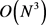，其中N为原始样本集的样本个数，且 ${n}_{1}+{n}_{2}=N$。DPS-SMO算法预选取的边界向量集里面的样本个数为2l，其中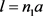。则DPS-SMO算法对边界向量集训练的计算复杂度为 $O\left(8{l}^{3}\right)$(13)

4. 基于距离配对排序支持向量预选取算法数值实验结果及比较

$K\left({x}_{1},{x}_{2}\right)=\mathrm{exp}\left(-\frac{{‖{x}_{1}-{x}_{2}‖}^{2}}{{r}^{2}}\right),$ (14)

4.1. 实验一Table 1. Comparison of classification performance in linear separable case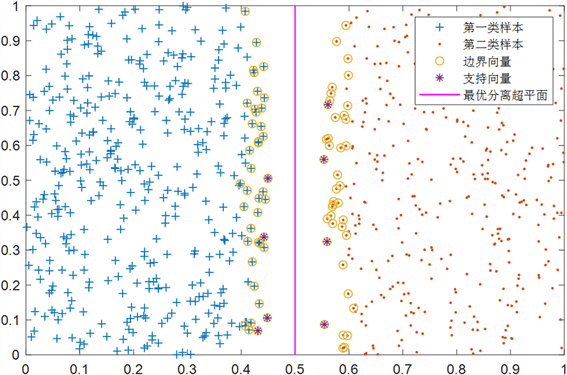Figure 2. Pre-selection of support vector by DPS-SMO in the case of linear separability

4.2. 实验二

$\left\{\begin{array}{l}{x}_{1}=\rho \mathrm{cos}\theta \\ {x}_{2}=\rho \mathrm{sin}\theta \end{array},\text{ }\text{ }\text{ }\text{ }\text{ }\text{ }\text{ }\theta \in \left[0,2\pi \right]$ (15)Table 2. Comparison of classification performance in nonlinear separable case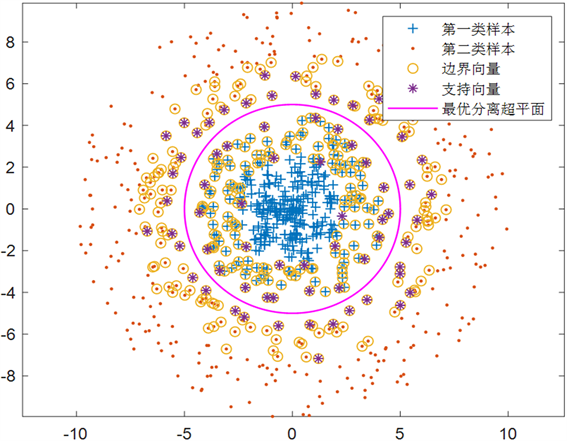Figure 3. Pre-selection of support vector by DPS-SMO in the case of nonlinear separability

4.3. 实验三

$a=36.4%.$Table 3. Comparison of classification performance under UCI data test

5. 结语

Pre-Selection of Support Vectors Base on Distance Pairing Sorting[J]. 应用数学进展, 2020, 09(02): 195-203. https://doi.org/10.12677/AAM.2020.92023

1. 1. Vapnik, V.N. (2000) The Nature of Statistical Learning Theory. Spring-Verlag, New York.

2. 2. Vapnik, V.N. (1999) An Overview of Statistical Learning Theory. IEEE Transactions on Neural Network, 10, 988-999. https://doi.org/10.1109/72.788640

3. 3. Yang, J., Yu, X. and Xie, Z.Q. (2011) A Novel Virtual Sample Generation Method Based on Gaussion Distribution. Knowledge-Based Systems, 24, 740-748. https://doi.org/10.1016/j.knosys.2010.12.010

4. 4. 李青, 焦李成, 周伟达. 基于向量投影的支持向量预选取[J]. 计算机学报, 2005, 28(2): 145-151.

5. 5. 琚旭, 王浩, 姚宏亮. 基于同心超球面分割的支持向量预抽取方法[J]. 计算机工程与应用, 2006(31): 55-56 + 83.

6. 6. 胡志军, 王鸿斌, 张惠斌. 基于距离排序的快速支持向量机分类算法[J]. 计算机应用与软件, 2013, 30(4): 85-87 + 100.

7. 7. 李庆, 胡捍英. 支持向量预选取的K边界近邻法[J]. 电路与系统学报, 2013, 18(2): 91-96.

8. 8. 邱静, 徐晓钟, 邓松, 王婷. 基于优化模糊C均值聚类选取相似日的燃气负荷预测[J]. 上海师范大学学报(自然科学版), 2017, 46(4): 560-566.

9. 9. 王石, 蒋宁宁, 杨舒卉. 基于压缩K近邻边界向量的支持向量预抽取算法[J]. 海军工程大学学报, 2018, 30(6): 74-79.